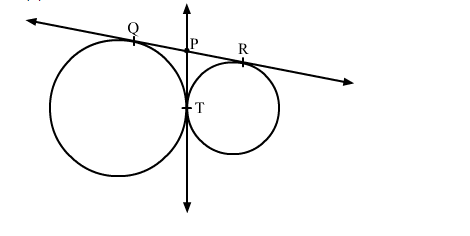# In the given figure, QR is a common tangent to the given circle, touching externally at the point T.`
Question:

In the given figure, QR is a common tangent to the given circle, touching externally at the point T. The tangent at T meets QR at P. If PT = 3.8 cm then the length of QR is

(a) 1.9 cm
(b) 3.8 cm
(c) 5.7 cm
(d) 7.6 cmSolution:

We know that tangent segments to a circle from the same external point are congruent.
Therefore, we have
PT = PQ = 3.8 cm and PT = PR = 3.8 cm
∴ QR = QP + PR = 3.8 + 3.8 = 7.6 cm
Hence, the correct answer is option (d)